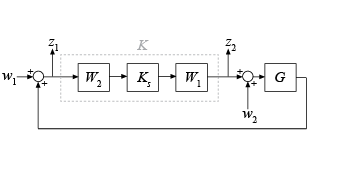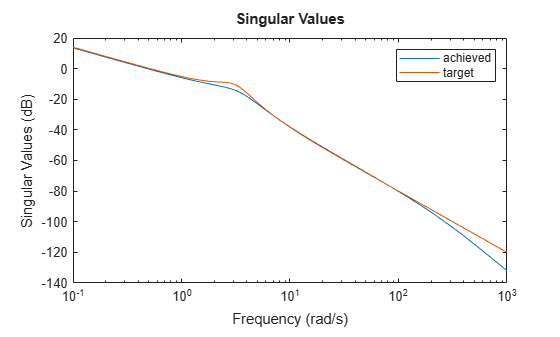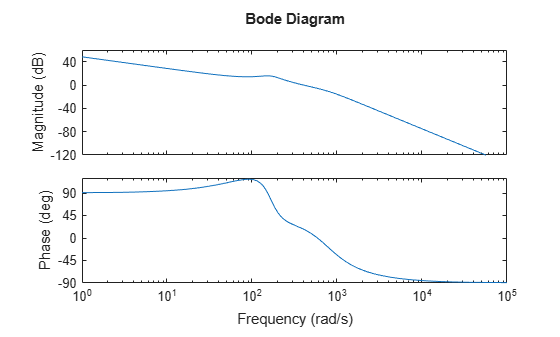# ncfsyn

Loop shaping design using Glover-McFarlane method

## Syntax

``[K,CL,gamma,info] = ncfsyn(G)``
``[K,CL,gamma,info] = ncfsyn(G,W1)``
``[K,CL,gamma,info] = ncfsyn(G,W1,W2)``
``[K,CL,gamma,info] = ncfsyn(G,W1,W2,tol)``

## Description

`ncfsyn` implements a method for designing controllers that uses a combination of loop shaping and robust stabilization as proposed in -. The function computes the Glover-McFarlane H normalized coprime factor loop-shaping controller K for a plant G with pre-compensator and post-compensator weights W1 and W2. The function assumes the positive feedback configuration of the following illustration.To specify negative feedback, replace G by –G. The controller Ks stabilizes a family of systems given by a ball of uncertainty in the normalized coprime factors of the shaped plant Gs = W2GW1. The final controller K returned by `ncfsyn` is obtained as K = W1KsW2.

````[K,CL,gamma,info] = ncfsyn(G)` computes the Glover-McFarlane H∞ normalized coprime factor loop-shaping controller `K` for the plant `G`, with W1 = W2 = I. `CL` is the closed-loop system from the disturbances w1 and w2 to the outputs z1 and z2. The function also returns the H∞ performance `gamma`, and a structure containing additional information about the result. ```

example

````[K,CL,gamma,info] = ncfsyn(G,W1)` computes the controller using the pre-compensator weight you specify in `W1`, with W2 = I.```
````[K,CL,gamma,info] = ncfsyn(G,W1,W2)` computes the controller using the specified pre-compensator weight `W1` and post-compensator weight `W2`.```

example

````[K,CL,gamma,info] = ncfsyn(G,W1,W2,tol)` specifies how tightly the performance `gamma` of the synthesized controller approximates the optimal achievable performance `gopt`. Increasing `tol` can help when the controller `K` has undesirable fast dynamics.```

## Examples

collapse all

Use `ncfsyn` to design a controller for the following plant.

`G = tf([1 5],[1 2 10]);`

Use weighting functions that yield a shaped plant `W1*G*W2` with high gain for disturbance attenuation below 0.1 rad/s, and low gain for good robust stability above about 5 rad/s. For this `G`, a pre-compensator weight alone is sufficient.

```W1 = tf(1,[1 0]); bodemag(W1*G) grid```Compute the controller.

`[K,CL,gamma,info] = ncfsyn(G,W1);`

The optimal cost `gamma` is related to the normalized coprime stability margin of the system by `1/gamma = ncfmargin(Gs,-Ks)`. (The minus sign is needed because `ncfmargin` assumes a negative-feedback loop, while `ncfsyn` computes a positive-feedback controller.)

```b = ncfmargin(info.Gs,-info.Ks); [gamma 1/b]```
```ans = 1×2 1.4521 1.4521 ```

Compare the achieved and target loop shapes.

```sigma(G*K,G*W1) legend('achieved','target')```In the controller returned by `ncfsyn`, some controller poles can become infinitely fast as the actual performance `gamma` approaches the optimal achievable performance `gopt`. To prevent this, check the controller poles and increase the `tol` argument if some poles are undesirably fast. `tol` sets how closely `gamma` of the synthesized controller approximates `gopt`.

Load `G`, a fourth-order plant.

```load("ncfsynTolPlant.mat") bode(G)```Use a weighting function that yields a shaped plant `W1*G` with high gain for disturbance attenuation below about 10 rad/s, and low gain for good robust stability above about 1000 rad/s.

```W1 = zpk(-130,0,0.6); bode(W1*G)```Design a controller using the default tolerance, `tol = 1e-3`. Examine the poles of the resulting controller.

```[K1,~,gamma1,info1] = ncfsyn(G,W1); damp(K1)```
``` Pole Damping Frequency Time Constant (rad/seconds) (seconds) 0.00e+00 -1.00e+00 0.00e+00 Inf -1.48e+02 + 1.60e+01i 9.94e-01 1.49e+02 6.76e-03 -1.48e+02 - 1.60e+01i 9.94e-01 1.49e+02 6.76e-03 -7.90e+02 + 8.01e+02i 7.02e-01 1.13e+03 1.27e-03 -7.90e+02 - 8.01e+02i 7.02e-01 1.13e+03 1.27e-03 -1.50e+05 1.00e+00 1.50e+05 6.66e-06 ```

This controller has a pole at around 150000 rad/s, much faster than any other dynamics in the controller. To reduce the frequency of this pole, try increasing the tolerance to 0.1.

```tol = 0.1; [K2,~,gamma2,info2] = ncfsyn(G,W1,[],tol); damp(K2)```
``` Pole Damping Frequency Time Constant (rad/seconds) (seconds) 0.00e+00 -1.00e+00 0.00e+00 Inf -1.50e+02 + 6.85e+00i 9.99e-01 1.50e+02 6.68e-03 -1.50e+02 - 6.85e+00i 9.99e-01 1.50e+02 6.68e-03 -6.58e+02 + 9.45e+02i 5.71e-01 1.15e+03 1.52e-03 -6.58e+02 - 9.45e+02i 5.71e-01 1.15e+03 1.52e-03 -1.77e+03 1.00e+00 1.77e+03 5.65e-04 ```

This time the highest-frequency pole is closer to the others. Compare the resulting performance values to confirm that the two controllers deliver similar performance.

`gamma1, gamma2`
```gamma1 = 2.1157 ```
```gamma2 = 2.3250 ```

## Input Arguments

collapse all

Plant, specified as a dynamic system model such as a state-space (`ss`) model. If `G` is a generalized state-space model with uncertain or tunable control design blocks, then `ncfsyn` uses the nominal or current value of those elements. `G` must have the same number of inputs and outputs.

Pre-compensator weight, specified as:

• Identity matrix `eye(N)`, where `N` is the number of inputs or outputs in `G`.

• SISO minimum-phase LTI model. In this case, `ncfsyn` uses the same weight for every loop channel.

• MIMO minimum-phase LTI model of the same I/O dimensions as `G`.

Select pre-compensator and post-compensator weights W1 and W2 such that the gain of the shaped plant Gs = W2GW1 is sufficiently high at frequencies where good disturbance attenuation is required, and sufficiently low at frequencies where good robust stability is required.

Post-compensator weight, specified as the identity matrix `eye(N)` or a SISO or MIMO LTI model. The considerations for specifying `W2` are the same as those for `W1`. To omit the post-compensator weight, set `W2 = []`.

Near-optimality tolerance, specified as a positive scalar value. `ncfsyn` returns a controller `K` with performance `gamma` that satisfies ```abs(gamma-gopt) < tol*gopt```, where `gopt` is the optimal performance (returned in `info`). Use `tol` to adjust the acceptable gap between `gamma` and `gopt`. A `tol` value that is too small can cause numerical difficulties and introduce fast poles in `K`. Increasing `tol` usually eliminates both issues. For an example, see Reduce Undesirable Fast Dynamics in Controller.

## Output Arguments

collapse all

H-optimal loop-shaping controller, returned as a state-space (`ss`) model with the same I/O dimensions as `G`. The optimal controller K = W1KsW2. See Algorithms.

Optimal closed-loop system from the disturbances w1 and w2 to the outputs z1 and z2, returned as a state-space model. The closed-loop system is given by:

`$\left[\begin{array}{c}I\\ K\end{array}\right]{\left(I-GK\right)}^{-1}\left[I,\text{\hspace{0.17em}}G\right].$`

H performance achieved with the controller `K`, returned as a positive scalar value greater than 1. The H performance is `hinfnorm(CL)`. The optimal controller Ks is such that the singular-value plot of the shaped loop Ls = W2GW1Ks optimally matches the target loop shape Gs to within a factor of `gamma`. However, for numerical reasons, `ncfsyn` generally returns a controller with slightly larger H performance than optimal. For the optimal achievable performance, see the `info` output argument.

`gamma` is related to the normalized coprime stability margin of the system by `gamma = 1/ncfmargin(Gs,-Ks)`. Thus, `gamma` gives a good indication of robustness of stability to a wide class of unstructured plant variations, with values in the range 1 < `gamma` < 3 corresponding to satisfactory stability margins for most typical control system designs.

Additional information about the controller synthesis, returned as a structure containing the following fields.

• `gopt` — Optimal performance achievable by H synthesis for the shaped plant. For numerical reasons, `ncfsyn` generally returns a controller with slightly larger H performance, which is returned in `gamma`. To adjust how tightly `ncfsyn` aims to make `gamma` match `gopt`, use the `tol` input argument. See Reduce Undesirable Fast Dynamics in Controller.

• `emax``nugap` robustness metric, `emax = 1/gopt` (see `gapmetric`)

• `Gs` — Shaped plant Gs = W2GW1

• `Ks` — Optimal controller for shaped plant `Gs`. The final controller is K = W1KsW2. See Algorithms for details.

## Tips

• While `ncfmargin` assumes a negative-feedback loop, the `ncfsyn` command designs a controller for a positive-feedback loop. Therefore, to compute the margin using a controller designed with `ncfsyn`, use ```[marg,freq] = ncfmargin(G,K,+1)```.

## Algorithms

The returned controller K = W1KsW2, where Ks is an optimal H controller that minimizes the H cost

`$\gamma \left({K}_{s}\right)={‖\left[\begin{array}{c}I\\ {K}_{s}\end{array}\right]{\left(I-{G}_{s}{K}_{s}\right)}^{-1}\left[I,{G}_{s}\right]‖}_{\infty }={‖\left[\begin{array}{c}I\\ {G}_{s}\end{array}\right]{\left(I-{K}_{s}{G}_{s}\right)}^{-1}\left[I,{K}_{s}\right]‖}_{\infty }.$`

The optimal performance is the minimal cost

`$\gamma :=\underset{{K}_{s}}{\mathrm{min}}\gamma \left({K}_{s}\right).$`

Suppose that Gs=NM–1, where N()*N() + M()*M() = I, is a normalized coprime factorization (NCF) of the weighted plant model Gs. Then, theory ensures that the control system remains robustly stable for any perturbation ${\stackrel{˜}{G}}_{s}$ to Gs of the form

`${\stackrel{˜}{G}}_{s}=\left(N+{\Delta }_{1}\right){\left(M+{\Delta }_{2}\right)}^{-1}$`

where Δ1, Δ2 are a stable pair satisfying

`${‖\left[\begin{array}{c}{\Delta }_{1}\\ {\Delta }_{2}\end{array}\right]‖}_{\infty }`

The closed-loop H-norm objective has the standard signal gain interpretation. Finally it can be shown that the controller, Ks, does not substantially affect the loop shape in frequencies where the gain of W2GW1 is either high or low, and will guarantee satisfactory stability margins in the frequency region of gain cross-over. In the regulator set-up, the final controller to be implemented is K=W1KsW2.

See McFarlane and Glover  for details.

## Compatibility Considerations

expand all

Not recommended starting in R2020b

 McFarlane, Duncan C., and Keith Glover, eds. Robust Controller Design using Normalized Coprime Factor Plant Descriptions. Vol. 138. Lecture Notes in Control and Information Sciences. Berlin/Heidelberg: Springer-Verlag, 1990. https://doi.org/10.1007/BFb0043199.

 McFarlane, D., and K. Glover, “A Loop Shaping Design Procedure using H Synthesis,” IEEE Transactions on Automatic Control, no. 6 (June 1992): pp. 759–69. https://doi.org/10.1109/9.256330.

 Vinnicombe, Glenn. “Measuring Robustness of Feedback Systems.” PhD Dissertation, University of Cambridge, 1992.

 Zhou, Kemin, and John Comstock Doyle. Essentials of Robust Control. Upper Saddle River, NJ: Prentice-Hall, 1998.

## SupportGet trial now